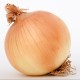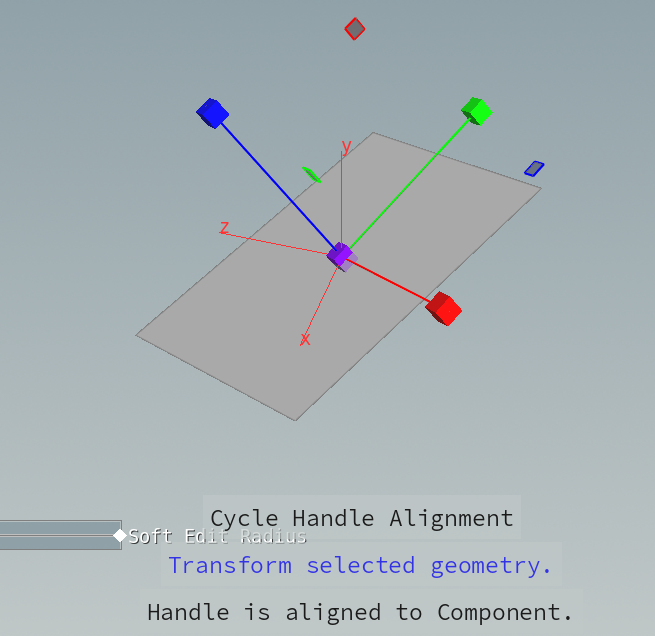# mk2 onion

onmk2

Expertise
Not Specified
Location
Not Specified
Website

## Recent Forum Posts

#### Procedural implementation of EditSop's "Align Handle: Component" ?June 2, 2019, 8:34 p.m.

I made a mistake in calc matrix, it must be not @P*AI, but AI*@P.

but the problem remains. How Houdini decide handle direction of EditSop?

```vector T = prim(0, "Tangent",   pointprims(0, @ptnum));
vector N = prim(0, "N",         pointprims(0, @ptnum));
vector B = prim(0, "BiTangent", pointprims(0, @ptnum));

vector R0 = set(T, N, B);
vector R1 = set(T, N, B);
vector R2 = set(T, N, B);

matrix3 A =  set(R0, R1, R2);
matrix3 AI = invert(A);

// scale shape in the coordinate system whose basis is Tangent, Normal, BiTangent
v@Pdash = AI * @P;
@Pdash = @Pdash * ch("./parmx");
@Pdash = @Pdash * ch("./parmy");
@Pdash = @Pdash * ch("./parmz");

// find result in the coordinate system whose basis is X:{1,0,0} Y:{0,1,0} Z:{0,0,1}
@P = A * @Pdash;
```

#### Procedural implementation of EditSop's "Align Handle: Component" ?June 1, 2019, 12:42 a.m.

By EditSop's “Align Handle: Component”, we can scale one primitive along component direction like below image.But I would like to scale many primitives along their component directions.
so procedural implementation is required.

I think I can do it if I scale it in Tangent Vector Space, and I wrote code.

```vector T = prim(0, "Tangent",   pointprims(0, @ptnum));
vector N = prim(0, "N",         pointprims(0, @ptnum));
vector B = prim(0, "BiTangent", pointprims(0, @ptnum));

vector R0 = set(T, N, B);
vector R1 = set(T, N, B);
vector R2 = set(T, N, B);

matrix3 A =  set(R0, R1, R2);
matrix3 AI = invert(A);

// scale shape in the "Tangent Vector Space" whose basis is Tangent, Normal, BiTangent
v@Pdash = @P * AI;
@Pdash = @Pdash * ch("./parmx");
@Pdash = @Pdash * ch("./parmy");
@Pdash = @Pdash * ch("./parmz");

// find result in the coordinate system whose basis is X:{1,0,0} Y:{0,1,0} Z:{0,0,1}
@P = @Pdash * A;
```

But the result of this is not what I want because it is far from EditSop's result.
You can see it in my hipnc file. please see the link at the bottom of post.

Perhaps the problem is that I can't understand how EditSop's “Align Handle: Component” decide each handle direction.

Could you help me how to implement procedurally EditSop's “Align Handle: Component”?

#### How to select primitives inside the edge loop which has been selected ?Jan. 21, 2019, 10:30 p.m.

How to select primitives inside the edge loop which has been selected ?

Hi

I have selected a edge loop like this,then I would like to select primitives like this.In blender, I can simply use “Select Loop Inner-Region” function.